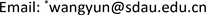﻿ 四元数矩阵特征值的Jacobi迭代 The Jacobi Iteration of Eigenvalue of Real Self-Adjoint Quaternion Matrices

Pure Mathematics
Vol.08 No.03(2018), Article ID:25073,5 pages
10.12677/PM.2018.83036

The Jacobi Iteration of Eigenvalue of Real Self-Adjoint Quaternion Matrices

Zhe Ouyang1, Yun Wang*

College of Information Sciences and Engineering, Shandong Agricultural University, Tai’an ShandongReceived: May 5th, 2018; accepted: May 18th, 2018; published: May 25th, 2018ABSTRACT

Quaternion matrix has a wide range of applications in the field of engineering technology, physics and computer science. In this paper, we describe the background and development of quaternion and quaternion matrices. Moreover some basic definitions and theorems of quaternion and quaternion matrices are demonstrated. Finally, we discuss the Jacobi iteration of right eigenvalues of real self-adjoint quaternion matrices based on the real-representation.

Keywords:Quaternion, Quaternion Matrices, Right Eigenvalue, Jacobi Iteration1. 引言

2. 基础知识

$q=a+bi+cj+dk\text{}a,b,c,d\in R$ (2.1.1)

$Q=\left\{a+bi+cj+dk|a,b,c,d\in R\right\}.$

${q}_{1}={a}_{1}+{b}_{1}i+{c}_{1}j+{d}_{1}k\in Q$${q}_{2}={a}_{2}+{b}_{2}i+{c}_{2}j+{d}_{2}k\in Q$ ，则两个四元数的相等、加法与乘法分别规定如下：

${q}_{1}={q}_{2}⇔{a}_{1}={a}_{2},{b}_{1}={b}_{2},{c}_{1}={c}_{2},{d}_{1}={d}_{2}$

${q}_{1}+{q}_{2}=\left({a}_{1}+{a}_{2}\right)+\left({b}_{1}+{b}_{2}\right)i+\left({c}_{1}+{c}_{2}\right)j+\left({d}_{1}+{d}_{2}\right)k$

$\begin{array}{c}{q}_{1}q{}_{2}=\left({a}_{1}{a}_{2}-{b}_{1}{b}_{2}-{c}_{1}{c}_{2}-{d}_{1}{d}_{2}\right)+\left({a}_{1}{b}_{2}+{b}_{1}{a}_{2}+{c}_{1}{d}_{2}-{d}_{1}{c}_{2}\right)i\\ +\left({a}_{1}{c}_{2}+{a}_{2}{c}_{1}+{b}_{2}{d}_{1}-{d}_{2}{b}_{1}\right)j+\left({a}_{1}{d}_{2}+{d}_{1}{a}_{2}+{b}_{1}{c}_{2}-{c}_{1}{b}_{1}\right)k\end{array}$

1) A的为复数的右特征值的集合 = ${A}_{\sigma }$ 的复特征值的集合；

2) A的右特征值的集合 = { ${a}^{-1}\lambda a$ | $0\ne a\in Q$$\lambda$${A}_{\sigma }$ 的复特征值}，

3. 自共轭实四元数矩阵的特征值

$A={A}_{0}+{A}_{1}i+A{}_{2}j+{A}_{3}k$

${A}^{\ast }=A$

${A}_{0}^{\text{T}}={A}_{0},\text{}{A}_{1}^{\text{T}}=-{A}_{1},\text{}{A}_{2}^{\text{T}}=-{A}_{2},\text{}{A}_{3}^{\text{T}}=-{A}_{3}$ (3.1.1)

$U+Vi+Wj+Gk$ ，其中 $U,V,W,G$ 均为实的n维列向量，则

$\left({A}_{0}+{A}_{1}i+{A}_{2}j+{A}_{3}k\right)\left(U+Vi+Wj+Gk\right)=\lambda \left(U+Vi+Wj+Gk\right)$ (3.1.2)

$\left(\begin{array}{cccc}{A}_{0}& -{A}_{1}& -{A}_{2}& -{A}_{3}\\ {A}_{1}& {A}_{0}& -{A}_{3}& {A}_{2}\\ {A}_{2}& {A}_{3}& {A}_{0}& -{A}_{1}\\ {A}_{3}& -{A}_{2}& {A}_{1}& {A}_{0}\end{array}\right)\left(\begin{array}{c}U\\ V\\ W\\ G\end{array}\right)=\lambda \left(\begin{array}{c}U\\ V\\ W\\ G\end{array}\right)$

$S=\left(\begin{array}{cccc}{A}_{0}& -{A}_{1}& -{A}_{2}& -{A}_{3}\\ {A}_{1}& {A}_{0}& -{A}_{3}& {A}_{2}\\ {A}_{2}& {A}_{3}& {A}_{0}& -{A}_{1}\\ {A}_{3}& -{A}_{2}& {A}_{1}& {A}_{0}\end{array}\right)$

${G}_{ij}\left(\theta \right)=\left(\begin{array}{ccccccccccc}1& & & & & & & & & & \\ & \ddots & & & & & & & & & \\ & & 1& & & & & & & & \\ & & & \mathrm{cos}\theta & & & & \mathrm{sin}\theta & & & \\ & & & & 1& & & & & & \\ & & & & & \ddots & & & & & \\ & & & & & & 1& & & & \\ & & & -\mathrm{sin}\theta & & & & \mathrm{cos}\theta & & & \\ & & & & & & & & 1& & \\ & & & & & & & & & \ddots & \\ & & & & & & & & & & 1\end{array}\right)$

${S}_{k}={G}_{k}{S}_{k-1}{G}_{k}^{\text{T}}\text{}\left(k=1,2,\cdot \cdot \cdot \right)$ (3.1.3)

4. 小结

The Jacobi Iteration of Eigenvalue of Real Self-Adjoint Quaternion Matrices[J]. 理论数学, 2018, 08(03): 273-277. https://doi.org/10.12677/PM.2018.83036

1. 1. 李文亮. 四元数矩阵[M]. 长沙: 国防科技大学出版社, 2002.

2. 2. 庄瓦金. 体上矩阵理论引导[M]. 北京: 科学出版社, 2006.

3. 3. Zhang, F.H. (1997) Quaternion and Matrices of Quaternion. Linear Algebra and its Applications, 251, 21-57. https://doi.org/10.1016/0024-3795(95)00543-9

4. 4. Huang, L.P. (2000) On Two Questions about Quaternion Matrices. Linear Algebra and its Applications, 318, 79-86. https://doi.org/10.1016/S0024-3795(00)00154-3

5. 5. 李桃生. 四元数矩阵的特征值与特征向量[J]. 华中师范学院学报, 1995, 29(4): 407-411.

6. 6. 黄礼平. 自共轭实四元数矩阵特征值的极值原理[J]. 数学研究与评论, 1997, 17(1): 101-104.

7. 7. Brualdi, R.A. (2003) The Spectral Theorem in Quaternions. Linear Algebra and its Applications, 371, 75-102. https://doi.org/10.1016/S0024-3795(02)00276-8

8. 8. 黄敬频, 陆云双. 自共轭四元数循环矩阵的特征值问题[J]. 数学的实践与认识, 2016(13): 251-257.

9. 9. 曾祥金, 张亮. 矩阵分析简明教程[M]. 北京: 科学出版社, 2010.

10. 10. 翟瑞彩, 谢伟松. 数值分析[M]. 天津: 天津大学出版社, 2000: 87-95.